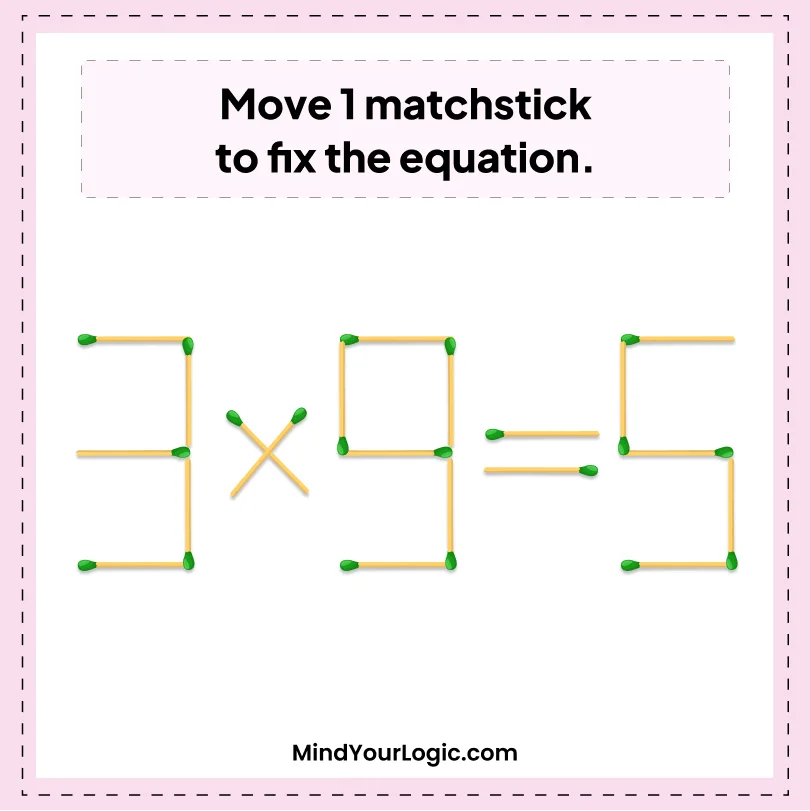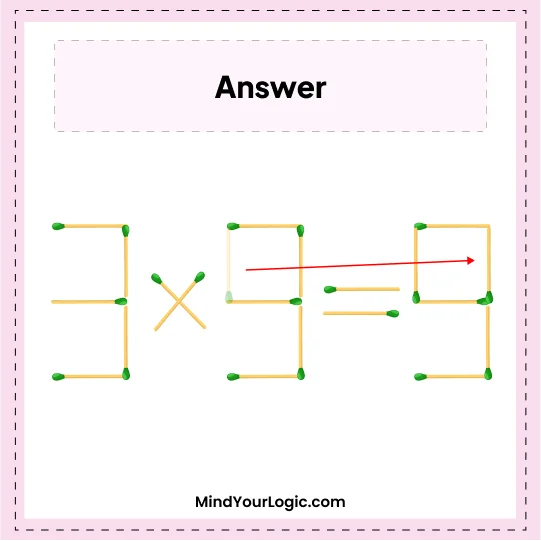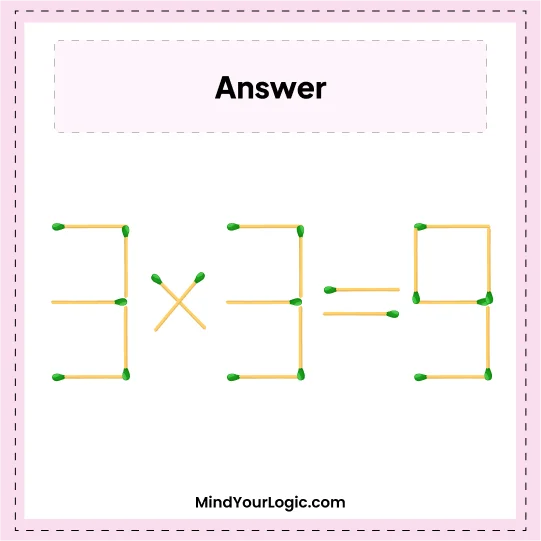# 3*9=5 Equation - Matchstick Puzzle

###### 7.Matchstick Puzzles
`Move 1 matchstick to fix the equation.`•

Explanation :

``` Move one matchstick from 9 and place it to 5, which becomes 3 and 9 respectively.
Now, the equation we get is 3*3 = 9```